×#### Thank you for registering.

One of our academic counsellors will contact you within 1 working day.

Click to Chat

1800-1023-196

+91-120-4616500

CART 0

• 0

MY CART (5)

Use Coupon: CART20 and get 20% off on all online Study Material

ITEM
DETAILS
MRP
DISCOUNT
FINAL PRICE
Total Price: Rs.

There are no items in this cart.
Continue Shopping• Complete Physics Course - Class 11
• OFFERED PRICE: Rs. 2,968
• View Details

Effect of Frequency and Intensity of Light in Photoelectric Effect

Table of Content

Effect of Collector’s Potential on Photoelectric Current

Effect of Intensity of Light

Effect of Frequency of Light

Photoelectric Current

Related Resources

The apparatus required for the experimental study of photoelectric effect as shown in figure. It consists of an evacuated glass tube ‘T’ fitted with two electrodes. Electrode ‘P’ known as emitter is coated with a photo-sensitive material. Light from a source, after passing through a quartz window ‘W’, is made to fall upon “P’. Collector C is kept at different positive or negative voltage with respect to P. The photoelectric effect can be studied with reference to the frequency and intensity of the incident light, number of photoelectrons emitted and their maximum energy. When proper positive potential is applied to collector C, all the  photoelectrons are attracted towards it and the maximum current recorded by the micro-ammeter gives an idea of the number of photo electrons.When negative potential is applied to the collector, only such electrons which have sufficient energy to overcome the negative potential may reach the collector. On making collector more negative, photoelectric current decreases and becomes zero at or lower than some specific negative potential. This minimum negative potential of the collector with respect to the photosensitive surface at which photoelectric current becomes zero is called stopping potential. According to the definition of stopping potential, electron on the surface of the photosensitive surface having maximum velocity,vmax , just reaches the collector plate overcoming stopping potential V0. In the process, the work eV0 done by it is at the cost of its kinetic energy, ( 1 / 2 ) m v2 max.Effect of Collector’s Potential on Photoelectric Current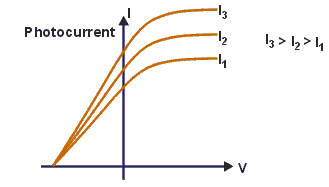Keeping the intensity and frequency of light from ‘S’ fixed, note the value of current in the circuit by changing potential of collector from positive to zero and to negative value. Plot a graph between the potential (along X-axis) and photoelectric current (along Y-axis). Two such curves, one for low intensity (i) and second for high intensity (ii) are shown in figure.

Each curves shows that the current changescontinuously with a change in potential. Beyond some positive potential of collector the current attains a saturation value while for a certain negative value ‘V0’ it is reduced to zero. ‘V0’ is called the stopping potential. Following conclusions can be drawn from above observations:

(a) Presence of current for zero value potential indicates that the electrons are ejected from the surface of emitter with some energy.

(b) A gradual change in number of electrons reaching the collector due to change in its potential indicates that the electrons are ejected with a variety of  velocities.

(c) Current is reduced to zero for some negative potential of collector indicating that there is some upper limit to the energy of electrons emitted.

(d) For any potential of collector the current in case of curve (b) is greater than that in case (a). This means the current depends upon the intensity of incident light.

(e) Both the curves meet at same point on X-axis indicating that stopping potential is independent of the intensity of light.

Effect of Intensity of Light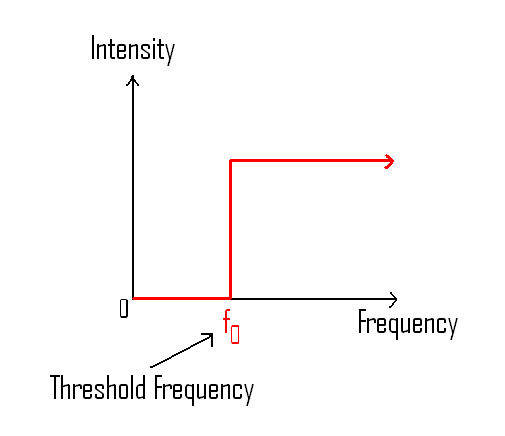Keeping potential of collector fixed note the value of current in the circuit for different intensity of light. A graph between intensity of light and photoelectric current is found to be a straight line indicating that the photoelectric current is directly proportional to the intensity of incident radiation.

Effect of Frequency of Light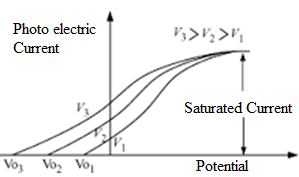Repeat this experiment as described in case (a) for different sources of frequencies f1,f2,and f3 giving same intensity of light. Three curves (i), (ii) and (iii) as shown in figure are obtained. The curves show that:

(i) Stopping potential depends upon the frequency of light. Greater the frequency of light greater is the stopping potential.

(ii) Saturation current is independent of frequency.

Note the values of stopping potential V01, V02, V03,....., for various frequencies f1, f2, f3,... respectively. Plot a graph between frequency (f) and the stopping potential. A straight line as shown in figure is obtained. The straight line has an intercept ‘f0’ there will be no current when collector is at zero potential. This is the minimum frequency capable of producing photoelectric effect and is called threshold frequency. Greater the frequency of incident light, greater the value of negative potential required to stop the current. Hence, maximum energy of the emitted electron depends upon the frequency of light.

Watch this Video for more reference

Photoelectric Current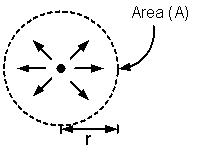Let P be the power of a point source of electromagnetic radiations, then intensity I at distance r from the source is given by

I = P/4πr2 (W/m2)

If A is the area of a metal surface on which radiations are incident, then the power received by the plate is

P' = IA = P/4πr2 (W)

If f is the frequency of radiation, then the energy of photon is given by

E = hf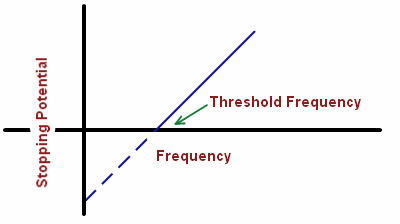The number of photons incident on the plate per second (called photon flux) is given by

Φ  = P' / E = [P/4πr2 × A / hf]

If f > f0 (threshold frequency) and photon efficiency of the metal plate is η%, then the number of photoelectrons emitted per second is given by

n = Φη/100 [P/4πr2 × A / hf]] η/100

Finally, the photocurrent i is given byWhere e is the charge of an electron (e = 1.6 × 10–19 J)Photo-electric current falls to zero for some negative potential of opposite electrode. This negative potential is called stopping potential.

Stopping potential is independent of intensity of incident light.

Stopping potential depend upon frequency of incident light.

Saturating current is independent of frequency of light.

Saturation current varies directly as the intensity of incident light.

Instantaneous photoelectric current varies directly as the intensity of incident light.

Photoelectric current stops below a particular frequency of incident light. The frequency is known as threshold frequency.

Work function is the characteristic of a photosensitive material.

Threshold frequency is equal to (1/h) times the work function.

Work function is equal to electronic charge times the intercept of (V0 – f) graph on V0 axis.

Electronic charge is equal to the multiplication of Planck’s constant and slope of (V0 – f) graph.

Photo-electric effect establishes the quantum nature of radiation. This can be considered to be a proof in favour of particle nature of light.

We further discuss some of the factors which have an impact on the photoelectric current.

In case the electroscope is negatively charged, it discharges slowly and the gold leaves fall down.

If the intensity or the amplitude of the ultra violet radiations is increased, it augments the emission of photoelectrons per second and hence the electrode discharges even more quickly. The number of photons released or emitted per second is proportional to the intensity of the radiation. This hold true in case of any metal.

In case the electroscope is positively charged, no loss of charge takes place. Since the zinc plate has a positive charge so the frère electrons present on it require much more energy to leave it.

Another point to be noted is that can visible light be used to cause photoelectric effect in zinc? Since eth visible light is below the threshold frequency of zinc, so it would not be able to cause any emission of electrons. Moreover, it is also not possible to increase the intensity of light to cause photo electricity. Availability of more light but of same frequency is not useful as it does not increase the energy of the light photons.

In order to escape the potential of the metal, it is necessary for an electron to do a certain amount of work. Hence, a certain amount of energy must be transferred to the electron by the incident radiation.

Different metals have different work functions. It is due to this reason that in metals with lower work function even a low frequency radiation fulfills the need by providing adequate energy to the electrons to escape. Similar is the case with metals having higher work function.

An electron and a photon have same wavelength. If p is the momentum of electron and E is the energy of photon. The magnitude of p/E in SI unit is

(a) 3.0108           (b) 3.3310-9

(c) 9.110-31        (d) 6.6410-34

Solution:

We know that, de-Broglie wave length for electron,

λ = h/p

or, p = h/λ

and E = hc/λ         (for photon)

Thus, p/E = 1/c

= 1/(3108 m/s) = 3.3310-9 s/m

Thus, from the above observation we conclude that, option (b) is correct.

Problem 2:

Photoelectric threshold of metallic silver is λ = 3800 Å. Ultra-violet light of λ = 2600 Å is incident on silver surface. Calculate

(i)     the value of work function in joule and in eV.

(ii)    maximum kinetic energy of the emitted photoelectrons.

(iii)    the maximum velocity of the photo electrons (mass of the electron = 9.11 x 10–31 kg)

Solution:

(i) I0 = 3800 Å

W = hf0 = h c/λ0 = 6.633 × 10–34 × 3 × 108 / 3800 × 10–10

J = 6.63 × 10–34 × 3 × 108 / 3800 × 10–10    J = 5.23 × 10–19        J = 3.27 eV

(ii)  Incident wavelength λ = 2600 Å

f = incident frequency = 3 × 108 / 2600 × 10–10 Hz

Then Tmax = hf – W0

hf = 6.63 × 1034 × 3 × 108 / 2600 × 10–10 = 6.63 × 3 / 2.6 × 10–19 J = 7.65 × 10–19 J = 4.78 eV

Tmax = hf – W0 = 4.78 eV – 3.27 eV = 1.51 eV.

(iii) Tmax = 1/2 mv2max

So, vmax = √2Tmax / m = √2 × 2.242 × 10–19 / 9.11 × 10–31 = 0.7289 × 106 ms–1

Problem 3:

Sun gives light at the rate of 1400 Wm–2 of area perpendicular to the direction of light. Assume λ(sun light) = 6000 Å. Calculate the

(a)    number of photons/sec arriving at 1m2 area at that part of the earth, and

(b)    number of photons emitted from the sun/sec assuming the average radius of Earth's orbit is 1.49 x 1011 m.

Solution:

I = 1400 W / m2;       I = 6000 A

(a) n/sec = A / E/photon = (1400 × 1) × (6000 × 10–10) / 6.63 × 10–34 × 3 × 108 = 4.22 × 1021

(b) n/sec = Power of Sun(W) / E/photon = I × (4R2) × (6000 × 10–10) / 6.63 × 10–34 × 3 × 108 = 1.178 × 1045

Problem 4:

An isolated hydrogen atom emits a photon of 10.2 eV. Calculate:

(a) momentum of photon emitted

(b)  recoil momentum of the atom

(c) kinetic energy of the recoil atom. Mass of proton = Mp = 1.67 x 10–27 kg.

Solution:

(a) momentum (p) = E/c

=> p = 10.2 × 1.6 × 10–19 / 3 × 108 = 5.44 × 10–27 kg m/s

(b) recoil momentum of atom = p (Law of conservation of momentum)

=> recoil momentum = 5.44 x 10–27 kg m/s

(c) KE = 1/2 mv2 (v = recoil speed of atom, m = mass of H-atom)

=> KE = 1/2 (p/m)2 = p2/2m .

=> KE = (5.44 × 10–27)2 / (2 × 1.67 × 10–27) = 8.86 × 10–27 JQuestion 1

Which of the following statements is true about a photon?

(a) A photon has zero mass and zero momentum.

(b) A photon has finite mass and a finite value of momentum

(c) A photon has zero mass but finite value of momentum

(d) A photon has finite mass but zero momentum.

Question 2

The photoelectric effect proved to be a problem for a wave model of light because

(a) the number of electrons ejected varied directly with the intensity of the light.

(b) the light intensity had no effect on the energy of the ejected electrons.

(c) the energy of the ejected electrons varied inversely with the intensity of the light.

(d) the energy of the ejected electrons varied directly with the intensity of the light.

Question 3

When the intensity of a light source is increased:

(a) the number of photons emitted by the source in unit time increases.

(b) the total energy of the photons emitted per unit time increases.

(c) more energetic photons are emitted.

(d) faster photons are emitted.

Question 4

If the wavelength of light in an experiment on photoelectric effect is doubled:

(a) the photoelectric emission will not take place.

(b) the photoelectric emission may or may not take place.

(c) the stopping potential will increase.

(d) The potential will decrease

Question 5

Which of the following are not dependent on the intensity of incident radiation in a photoelectric experiment?

(a) amount of photoelectric current.

(b) stopping potential to reduce the photoelectric current to zero.

(c) work function of the surface.

(d) maximum kinetic energy of photoelectrons.Q.1
Q.2
Q.3
Q.4
Q.5

c

b

a, b

b, d

b,c, and d

Related Resources

You might like to de-Broglie Wave.

For getting an idea of the type of questions asked, refer the  Previous Year Question Papers.### Course Features

• 101 Video Lectures
• Revision Notes
• Previous Year Papers
• Mind Map
• Study Planner
• NCERT Solutions
• Discussion Forum
• Test paper with Video Solution